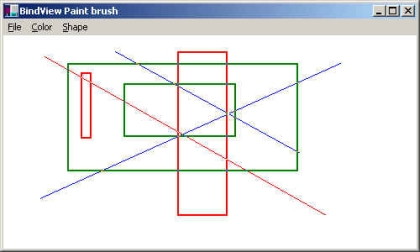The article is the paintbrush application, which demonstrates the different aspects of C# language and certain namespaces. The concepts like EventHandling and class designs are also present.I developed the application in VS .NET Beta1. The application is completely hand-coded so could even be compiled thru command line w/o much hassles.

Some of code snippet is here. Download zip file for full version.

public class Rectangle : Shape
{
protected Point m_Start;
protected Point m_End;
public Rectangle(Point start, Point end, Color fgColor)
{
m_Start = start;
m_End = end;
m_Color = fgColor;
}
public override void Draw(Form canvas)
{
if (canvas == null)
{
return;
}
InitializeGraphics(canvas);
Point startPoint = canvas.PointToScreen(m_Start);
Point endPoint = canvas.PointToScreen(m_End);
MainForm mainForm = (MainForm)canvas;
Color bgColor = GetBackgroundColor(m_Color);
Size rectSize =
new Size(m_End.X - m_Start.X, m_End.Y - m_Start.Y);
System.Drawing.Rectangle rectToDraw =
new System.Drawing.Rectangle(startPoint, rectSize);
ControlPaint.DrawReversibleFrame(rectToDraw, bgColor, FrameStyle.Thick);
}
}